## Math 480 Course Notes -- May 28, 1996

### Bivariate distributions

Recall that at the end of the last lecture we had started to discuss joint probability functions of two (or more) random variables. With two random variables X and Y, we define joint probability functions as follows:

For discrete variables, we let p(i,j) be the probability that X=i and Y=j. This give a function p, called the joint probability function of X and Y that is defined on (some subset of) the set of pairs of integers and such that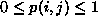for all i and j and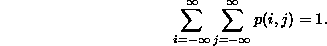When we find it convenient to do so, we will set p(i,j)=0 for all i and j outside the domain we are considering.

For continuous variables, we define the joint probability density function p(x,y) on (some subset of) the plane of pairs of real numbers. We interpret the function as follows: p(x,y)dxdy is (approximately) the probability that X is between x and x+dx and Y is between y and y+dy (with error that goes to zero faster than dx and dy as they both go to zero). Thus, p(x,y) must be a non-negative valued function with the property that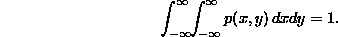As with discrete variables, if our random variables always lie in some subset of the plane, we will define p(x,y) to be 0 for all (x,y) outside that subset.

We take one simple example of each kind of random variable. For the discrete random variable, we consider the roll of a pair of dice. We assume that we can tell the dice apart, so there are thirty-six possible outcomes and each is equally likely. Thus our joint probability function will be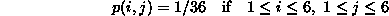and p(i,j)=0 otherwise.

For our continuous example, we take the example mentioned at the end of the last lecture: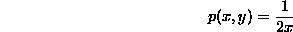for (x,y) in the triangle with vertices (0,0), (2,0) and (2,2), and p(x,y)=0 otherwise. We checked last time that this is a probability density function (its integral is 1).

### Marginal distributions

Often when confronted with the joint probability of two random variables, we wish to restrict our attention to the value of just one or the other. We can calculate the probability distribution of each variable separately in a straightforward way, if we simply remember how to interpret probability functions. These separated probability distributions are called the marginal distributions of the respective individual random variables.

Given the joint probability function p(i,j) of the discrete variables X and Y, we will show how to calculate the marginal distributions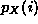of X and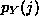of Y. To calculate, we recall thatis the probability that X=i. It is certainly equal to the probability that X=i and Y=0, or X=i and Y=1, or .... In other words the event X=i is the union of the events X=i and Y=j as j runs over all possible values. Since these events are disjoint, the probability of their union is the sum of the probabilities of the events (namely, the sum of p(i,j)). Thus:Likewise,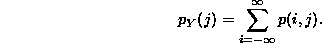Make sure you understand the reasoning behind these two formulas!

An example of the use of this formula is provided by the roll of two dice discussed above. Each of the 36 possible rolls has probability 1/36 of occurring, so we have probability function p(i,j) as indicated in the following table: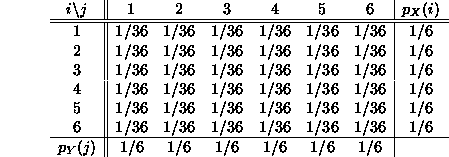The marginal probability distributions are given in the last column and last row of the table. They are the probabilities for the outcomes of the first (resp second) of the dice, and are obtained either by common sense or by adding across the rows (resp down the columns).

For continuous random variables, the situation is similar. Given the joint probability density function p(x,y) of a bivariate distribution of the two random variables X and Y (where p(x,y) is positive on the actual sample space subset of the plane, and zero outside it), we wish to calculate the marginal probability density functions of X and Y. To do this, recall that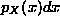is (approximately) the probability that X is between x and x+dx. So to calculate this probability, we should sum all the probabilities that both X is in [x,x+dx] and Y is in [y,y+dy] over all possible values of Y. In the limit as dy approaches zero,this becomes an integral: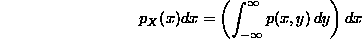In other words,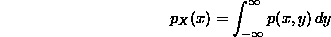Similarly,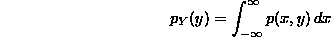Again, you should make sure you understand the intuition and the reasoning behind these important formulas.for (x,y) in the triangle with vertices (0,0), (2,0) and (2,2), and p(x,y)=0 otherwise, and compute its marginal density functions. The easy one is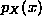so we do that one first. Note that for a given value of x between 0 and 2, y ranges from 0 to x inside the triangle: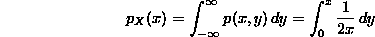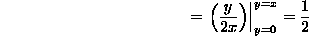if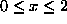, andotherwise. This indicates that the values of X are uniformly distributed over the interval from 0 to 2 (this agrees with the intuition that the random points occur with greater density toward the left side of the triangle but there is more area on the right side to balance this out).

To calculate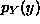, we begin with the observation that for each value of y between 0 and 2, x ranges from y to 2 inside the triangle: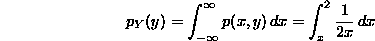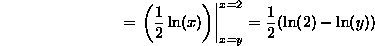if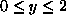and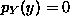otherwise. Note thatapproaches infinity as y approaches 0 from above, andapproaches 0 as y approaches 2. You should check that this function is actually a probability density function on the interval [0,2], i.e., that its integral is 1.

### Functions of two random variables

Frequently, it is necessary to calculate the probability (density) function of a function of two random variables, given the joint probability (density) function. By far, the most common such function is the sum of two random variables, but the idea of the calculation applies in principle to any function of two (or more!) random variables.

The principle we will follow for discrete random variables is as follows: to calculate the probability function for F(X,Y), we consider the events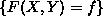for each value of f that can result from evaluating F at points of the sample space of (X,Y). Since there are only countably many points in the sample space, the random variable F that results is discrete. Then the probability function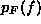is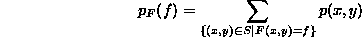This seems like a pretty weak principle, but it is surprisingly useful when combined with a little insight (and cleverness).

As an example, we calculate the distribution of the sum of the two dice. Since the outcome of each of the dice is a number between 1 and 6, the outcome of the sum must be a number between 2 and 12. So for each f between 2 and 12: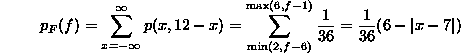A table of the probabilities of various sums is as follows: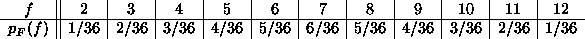The "tent-shaped" distribution that results is typical of the sum of (independent) uniformly distributed random variables.

For continuous distributions, our principle will be a little more complicated, but more powerful as well. To enunciate it, we recall that to calculate the probability of the event F<f, we integrate the pdf of F from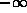to f: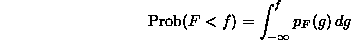Conversely, to recover the pdf of F, we can differentiate the resulting function: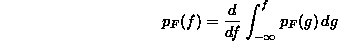(this is simply the first fundamental theorem of calculus). Our principle for calculating the pdf of a function of two random variables F(X,Y) will be to calculate the probabilities of the events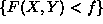(by integrating the joint pdf over the region of the plane defined by this inequality), and then to differentiate with respect to f to get the pdf.

We apply this principle to calculate the pdf of the sum of the random variables X and Y in our example:for (x,y) in the triangle T with vertices (0,0), (2,0) and (2,2), and p(x,y)=0 otherwise. Let Z=X+Y. To calculate the pdf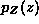, we first note that for any fixed number z, the region of the plane where Z<z is the half plane below and to the left of the line y=z-x. To calculate the probability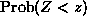, we must integrate the joint pdf p(x,y) over this region. Of course, for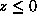, we get zero since the half plane z<0 has no points in common with the triangle where the pdf is supported. Likewise, since both X and Y are always between 0 and 2 the biggest the sum can be is 4. Therefore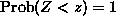for all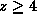.

For z between 0 and 4, we need to integrate 1/2x over the intersection of the half-plane x+y<z and the triangle T. The shape of this intersection is different, depending upon whether z is greater than or less than 2: If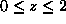, the intersection is a triangle with vertices at the points (0,0), (z/2,z/2) and (z,0). In this case, it is easier to integrate first with respect to x and then with respect to y, and we can calculate: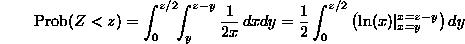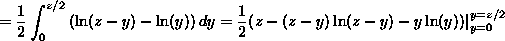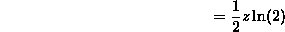And since the (cumulative) probability that Z<z is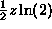for 0<z<2, the pdf over this range is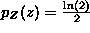.

The calculation of the pdf for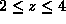is somewhat trickier because the intersection of the half-plane x+y<z and the triangle T is more complicated. The intersection in this case is a quadrilateral with vertices at the points (0,0), (z/2,z/2), (2,z-2) and (2,0). We could calculateby integrating p(x,y) over this quadrilateral. But we will be a little more clever: Note that the quadrilateral is the "difference" of two sets. It consists of points inside the triangle with vertices (0,0), (z/2,z/2), (z,0) that are to the left of the line x=2. In other words it is points inside this large triangle (and note that we already have computed the integral of 1/2x over this large triangle to be) that are not inside the triangle with vertices (2,0), (2,z-2) and (z,0). Thus, for, we can calculateas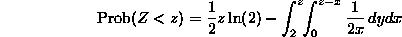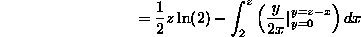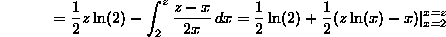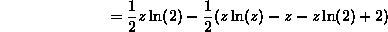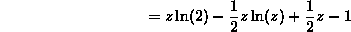To get the pdf for 2<z<4, we need only differentiate this quantity, to get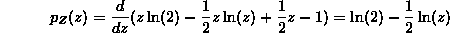Now we have the pdf of Z = X+Y for all values of z. It is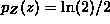for 0<z<2, it is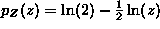for 2<z<4 and it is 0 otherwise. It would be good practice to check that the integral ofis 1.

### Conditional Probability

In our study of stochastic processes, we will often be presented with situations where we have some knowledge that will affect the probability of whether some event will occur. For example, in the roll of two dice, suppose we already know that the sum will be greater than 7. This changes the probabilities from those that we computed above. The event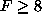has probability (5+4+3+2+1)/36=15/36. So we are restricted to less than half of the original sample space. We might wish to calculate the probability of getting a 9 under these conditions. The quantity we wish to calculate is denoted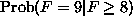, read "the probability that F=9 given that".

In general to calculate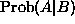for two events A and B (it is not necessary that A is a subset of B), we need only realize that we need to compute the fraction of the time that the event B is true, it is also the case the A is true. In symbols, we have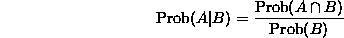For our dice example (noting that the event F=9 is a subset of the event), we get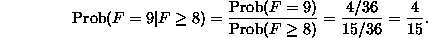As another example (with continuous probability this time), we calculate for our 1/2x on the triangle example the conditional probabilities: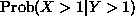as well as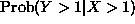(just to show that the probabilities of A given B and B given A are usually different).

First. This one is easy! Note that in the triangle with vertices (0,0), (2,0) and (2,2) it is true that Y>1 implies that X>1. Therefore the events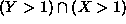and Y>1 are the same, so the fraction we need to compute will have the same numerator and denominator. Thus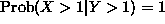.

Forwe actually need to compute something. But note that Y>1 is a subset of the event X>1 in the triangle, so we get: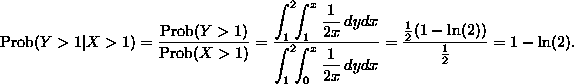Two events A and B are called independent if the probability of A given B is the same as the probability of A (with no knowledge of B) and vice versa. The assumption of independence of certain events is essential to many probabilistic arguments. Independence of two random variables is expressed by the equations: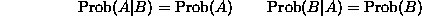and especially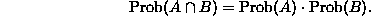Two random variables X and Y are independent if the probability that a<X<b remains unaffected by knowledge of the value of Y and vice versa. This reduces to the fact that the joint probability (or probability density) function of X and Y "splits" as a product: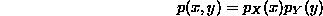of the marginal probabilities (or probability densities). This formula is a straightforward consequence of the definition of independence and is left as an exercise.

### Homework

1. Let p(x,y) be the uniform joint probability density on the unit disk, i.e.,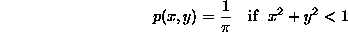and p(x,y)=0 otherwise. Calculate the pdf of X+Y. Also find the expected value and variance of X+Y.

2. Suppose X and Y are independent random variables, each distributed according to the exponential distribution with parameter. Find the joint pdf of X and Y (easy). Find the pdf of X+Y. Also find the mean and variance of X+Y.

3. Prove that two random variables are independent if and only if their joint probability (density) function is the product of the marginal probability (density) functions.

Dennis DeTurck
Mon Jun 10 17:32:18 EDT 1996